# Mathematics - Signals and Systems - Z Transform

in StemSocial2 months ago[Image1]

## Introduction

Hey it's a me again @drifter1!

Today we continue with my mathematics series about Signals and Systems in to get into the Z Transform.

So, without further ado, let's dive straight into it!

## Getting into the Z Transform

Similar to how the Laplace Transform has been developed as an generalization of the continuous-time Fourier Transform, the Z Transform is the corresponding equivalent for discrete-time. In discrete-time Fourier analysis, complex exponentials where used as basic building blocks for signals.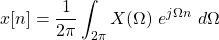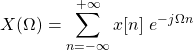Those exponentials of the form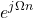are now replaced with the more general form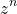, where z is a complex number.

As with the continuous-time Fourier Transform and Laplace Transform, a similar close relationship also exists in the case of the Z Transform and discrete-time Fourier Transform. To get even more specific, in the case where z = e , which is basically a magnitude of unity (as we will see in the ROC section), the Z Transform recudes to the Fourier Transform. So, similar to the Laplace Transform, the Z Transform can again be viewed as some kind of exponential weighting. And because of this exponential weighting sequences/signals that don't converge using the Fourier Transform may converge with the Z Transform, thus allowing for more signals and systems to be analyzed.

## Z Transform

Mathematically, the Z Transform is defined as: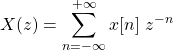And denoting the Transform by its letter Z, the Z Transform is basically the following function: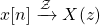Using the Z Transform, the impulse response (system response for a complex exponential) can be defiend as follows: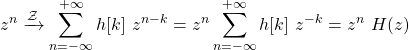In this previous equation the following substitution has been made: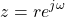which can also be used to relate the Fourier and Z Transform, as follows: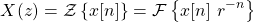Thus, its now clear as day that the Z Transform applies exponential weighting of the form r-n, allowing this Transform to converge in cases where the Fourier Transform might not.

### Example

Let's get into a simple example, to understand the concept better...

Consider the following commonly used signal: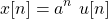Its a known case of the Fourier Transform and gives the following result: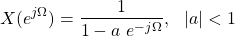Let's note that X(Ω) is the same as X(e ), and so referring to the Fourier Transform.

Substituting the corresponding z, yields the following result: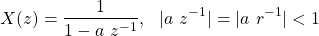In the case of the Z Transform, the conditions are far more important and play a key role in the so called Region of Convergence (ROC) of the Transform. More on that later on, but let's quickly summarize the Z Transform for the case of the exponential signal: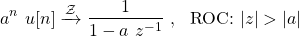## Region of Convergence (ROC)

The algebraic expression of the Z Transform can be similar for different signals, and so the range of values of z, referred to as the region of convergence (ROC), for which the given expression is valid, is also important to specify.

Similar to the Laplace Transform, the Z Transform can again by described as a ratio of polynomials in z.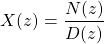As such, its convenient to describe the signal by the location of poles (roots of the denominator polynomial) and zeros (roots of the numerator polynomial) in the complex plane or z-plane. This complex z-plane is reduced to a unit circle (circle of radius 1) concentric with the origin, when the Z Transform reduces to the Fourier Transform. In the case of the Laplace Transform this was a little different as the imaginary axis in the s-plane played that role. Either way, the pole-zero pattern in the z-plane can be used to specify the algebraic expression for the Z Transform, but its also important to implicitly or explicitly indicate the ROC.

The z-plane and the corresponding unit circle are visualized below.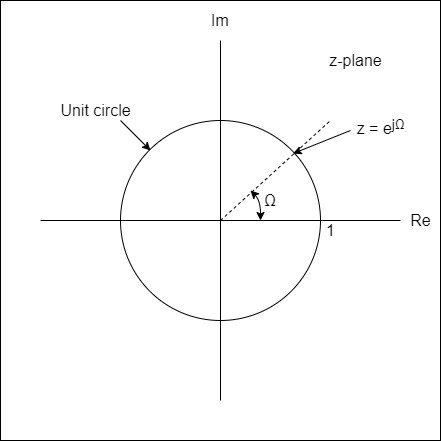### Example (Continued)

Well, let's get back to the previous example, to see how the ROC and the pole-zero pattern is visualized.In the case of this exponential signal, there are no zeros (roots of the numerator), and the pole is basically defining a concentric circle of radius a. Thus, the whole region around that circle is the ROC of the signal. Below is a visualization of the ROC and pole-zero pattern in the z-plane.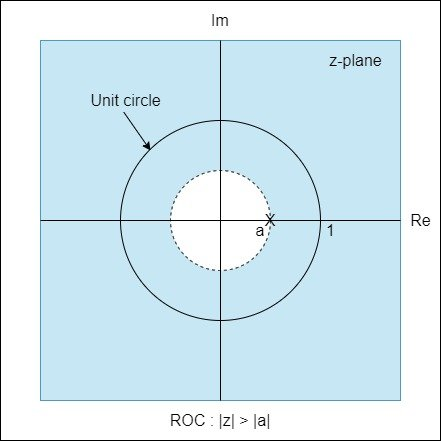Its easy to notice that the signal still converges even for values of |a| > 1 (outside of the unit circle), which don't converge in the case of the Fourier Transform.

The properties of the Z Transform and its ROC will be covered in-depth next time!

## Inverse Z Transform

Lastly, let's quickly mention how the inverse Z Transform is calculated.

Mathematically, the inverse Z Transform equation is as follows: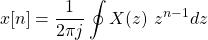Of course, Transform tables are used, and such contour integrals don't have to be calculated.

### Exercise for the viewer

Try to come up with this equation starting of with the notation: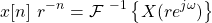and substituting z = re .

## RESOURCES:

### Images

Block diagrams and other visualizations were made using draw.io and GeoGebra

## Final words | Next up

And this is actually it for today's post!

Next time we will get into the various properties of the Z Transform...

See Ya!Keep on drifting!